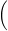# Percent Error and Percent Difference

## Percent Error

When comparing an experimental result to a value determined by theory or to an accepted known value (like
g = 9.8 m/s2)
we determine the difference between the experimental value and the theoretical value as a percentage of the theoretical value. In the definition below, "theoretical" is the value that is determined from theory (i.e., calculated from physics equations) or taken as a known or accepted value like g.
( 1 )
% error =
 |theoretical − experimental| theoretical
× 100%

## Percent Difference

When wanting to compare two values that are both determined by experimentation, we don't have a "correct" or accepted value to which to compare, so we simply look at how different the two values are as a percentage of their average value:
( 2 )
% difference =
|value 1 − value 2|value 1 + value 2 2× 100%.